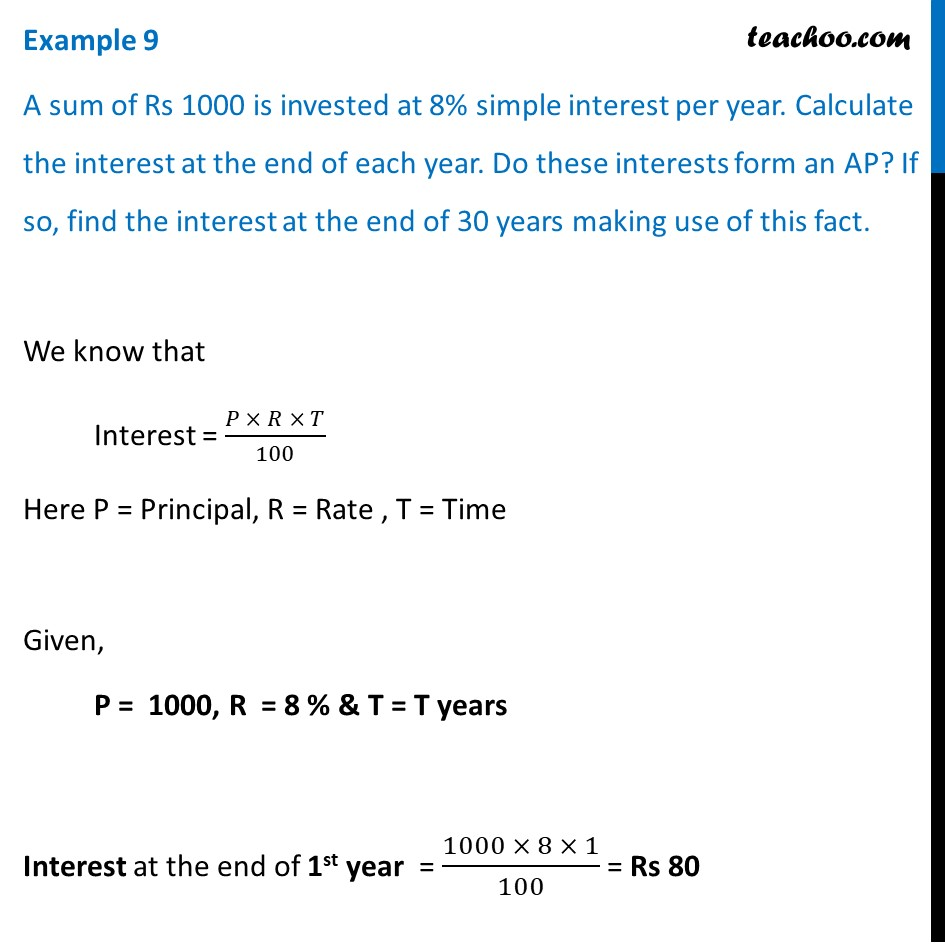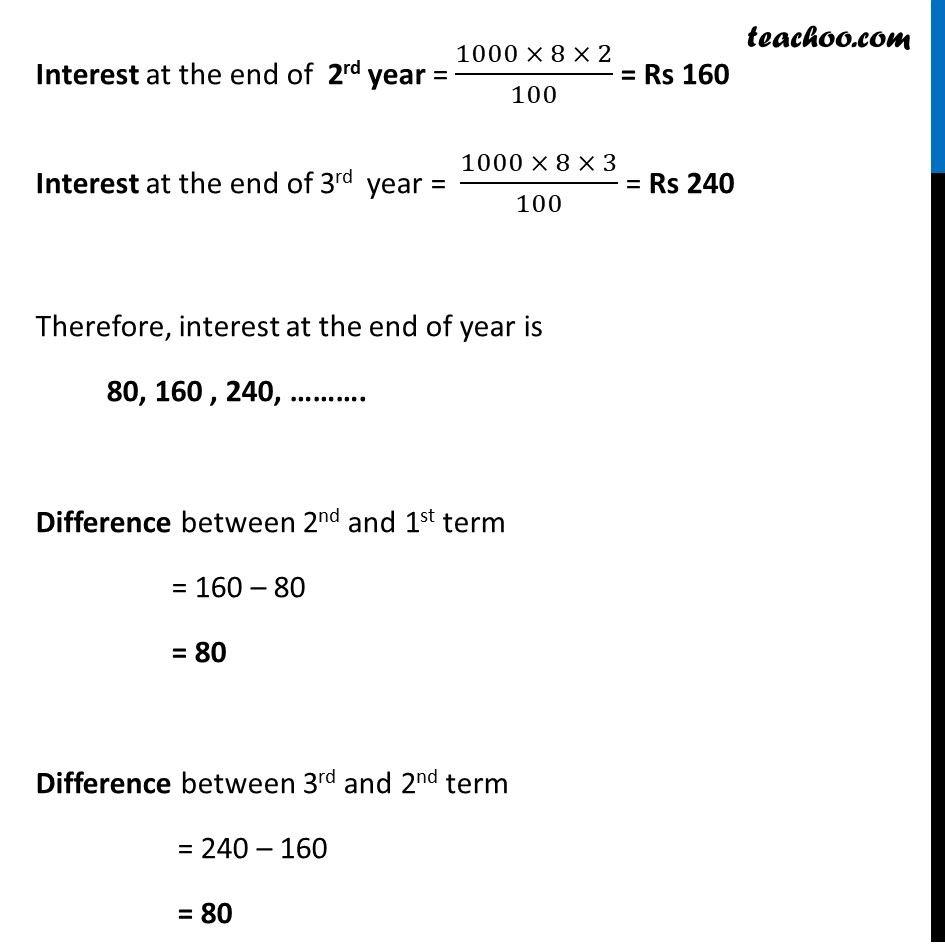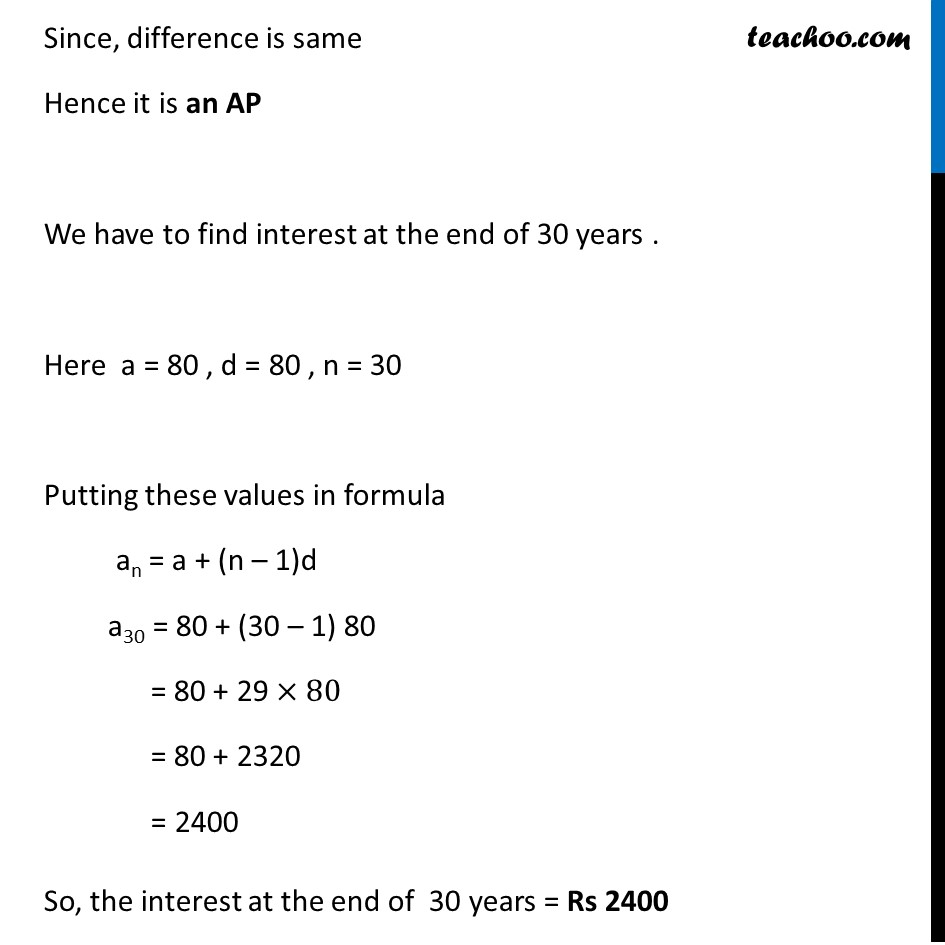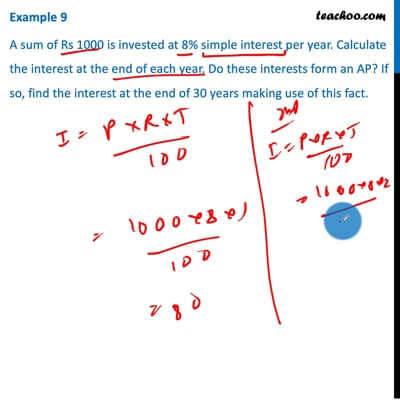Examples

Chapter 5 Class 10 Arithmetic Progressions
Serial order wiseThis video is only available for Teachoo black users

Solve all your doubts with Teachoo Black (new monthly pack available now!)

### Transcript

Example 9 A sum of Rs 1000 is invested at 8% simple interest per year. Calculate the interest at the end of each year. Do these interests form an AP? If so, find the interest at the end of 30 years making use of this fact. We know that Interest = (𝑃 × 𝑅 × 𝑇)/100 Here P = Principal, R = Rate , T = Time Given, P = 1000, R = 8 % & T = T years Interest at the end of 1st year = (1000 × 8 × 1)/100 = Rs 80 Interest at the end of 2rd year = (1000 × 8 × 2)/100 = Rs 160 Interest at the end of 3rd year = (1000 × 8 × 3)/100 = Rs 240 Therefore, interest at the end of year is 80, 160 , 240, ………. Difference between 2nd and 1st term = 160 – 80 = 80 Difference between 3rd and 2nd term = 240 – 160 = 80 Since, difference is same Hence it is an AP We have to find interest at the end of 30 years . Here a = 80 , d = 80 , n = 30 Putting these values in formula an = a + (n – 1)d a30 = 80 + (30 – 1) 80 = 80 + 29 ×80 = 80 + 2320 = 2400 So, the interest at the end of 30 years = Rs 2400